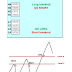## Top Ad unit 728 × 90

Camarilla is a trading method used for doing intraday trading in various markets like Forex, Equities, Futures, Commodities. This suggests that today's intraday support and resistance can be predicted using yesterday's volatility. Camarilla Equation produces 8 levels from yesterday's high, low and close. These levels are split into two groups, numbered 1 to 4. The pattern formed by the 8 levels is broadly symmetrical, and the most important levels are the 'L3', 'L4' and 'H3', 'H4' levels. While day trading, traders look for the market to reverse if it hits an 'L3' or 'H3' level. They would then open a position AGAINST the trend, using a stop loss somewhere before the associated 'L4' or 'H4' level.
Detailed process is explained below.
The another way to day trade with the Camarilla Equation is to regard the 'H4' and 'L4' levels as 'breakout' levels - in other words to go WITH the trend if prices push through either the H4 or L4 level. This essentially covers all the bases - Day Trading within the H3 and L3 levels enables you to capture all the wrinkles that intraday market movement throws up, and the H4 - L4 breakout plays allow the less experienced trader to capitalise on relatively low risk sharp powerful movements.

What is the method to calculate the simple camarilla levels?
C = Previous Day Close
H = Previous Day High
L = Previous Day Low
H4 = [0.55*(H-L)]+C
H3 = [0.275*(H-L)]+C
H2 = [0.183*(H-L)]+C
H1 = [0.0916*(H-L)]+C
L1 = C-[0.0916*(H-L)]
L2 = C-[0.183*(H-L)]
L3 = C-[0.275*(H-L)]
L4 = C-[0.55*(H-L)]
How to trade based on Simple Camarilla Levels ?
Once the camarilla levels from L4 – H4 are found, trading is done based on the open price of the stock or underlying. Instead of open price, we can also take the WAP (Weighted Average Price) of the first 5 min of market open.
Now depending on the open price, we consider below four scenarios:
Scenario 1: Open price is between H3 and L3
- To enter long position (i.e. To Buy)
o Wait for the price to go below L3 and then when it moves back above L3,
o Stoploss should be placed when price moves below (L3+L4)/2.
o Targets : Target1 = H1, Target2 = H2, Target3 = H3
- To enter short position (i.e. To Sell)
o Wait for the price to go above H3 and then when it moves back below H3,sell
or go short.
o Stoploss should be placed when price moves above (H4+H3)/2.
o Targets : Target1 = L1, Target2 = L2, Target3 = L3
Scenario 2: Open price is between H3 and H4
- To enter long position (i.e. To Buy)
o Wait for the price to go above H4. As soon as price moves above H4 buy.
o Stoploss should be placed when price moves below (H4+H3)/2.
o Targets : Target1 = 0.5%, Target2 = 1%, Target3 = 1.5%
- To enter short position (i.e. To Sell)
o Wait for the price to go below H3. As soon as the price moves below H3, sell
or go short.
o Stoploss should be placed when price moves above (H4+H3)/2.
o Targets : Target1 = L1, Target2 = L2, Target3 = L3
Scenario 3: Open price is between L3 and L4
- To enter long position (i.e. To Buy)
o Wait for the price to go above L3. As soon as price moves above L3 buy.
o Stoploss should be placed when price moves below (L4+L3)/2.
o Targets : Target1 = H1, Target2 = H2, Target3 = H3
- To enter short position (i.e. To Sell)
o Wait for the price to go below L4. As soon as the price moves below L4, sell
or go short.
o Stoploss should be placed when price moves above (L3+L4)/2.
o Targets : Target1 = 0.5%, Target2 = 1%, Target3 = 1.5%
Scenario 4: Open price is above H4
- To enter long position (i.e. To Buy)
o NA. Buying is not suggested in such scenarios.
- To enter short position (i.e. To Sell)
o Wait for the price to go below H3. As soon as the price moves below H3, sell
or go short.
o Stoploss should be placed when price moves above (H4+H3)/2.
o Targets : Target1 = L1, Target2 = L2, Target3 = L3
Scenario 5: Open price is below L4
- To enter long position (i.e. To Buy)
o Wait for the price to go above L3. As soon as price moves above L3 buy.
o Stoploss should be placed when price moves below (L4+L3)/2.
o Targets : Target1 = H1, Target2 = H2, Target3 = H3.
- To enter short position (i.e. To Sell)
o NA. Selling is not suggested in such case.
A simplification is to calculate profit targes with ratio stop loss 1: 1
Filter when distance between L4 - L3 and H3 .H4 is below of 20 pips do not trade GBP/USD
EUR/USD 16 pips, USD/JPY 20 pips.
Camarilla pivot is suitable for trading with Martingala.
See examples in pictures:

Intraday Trading Using CamarillaReviewed by learn forex trading on June 01, 2017 Rating: 5